Anzeige

22. Mar 2023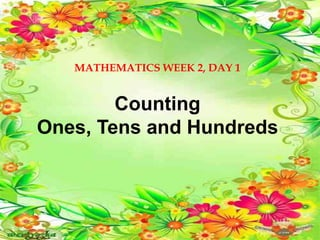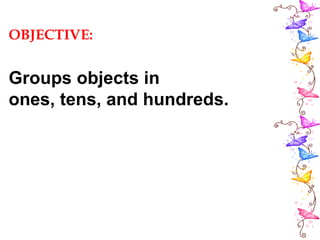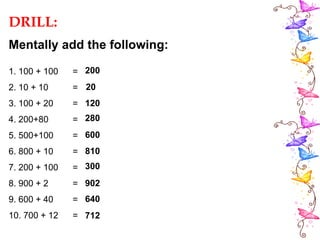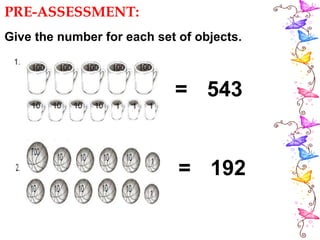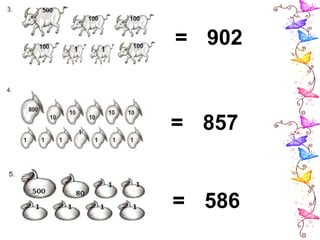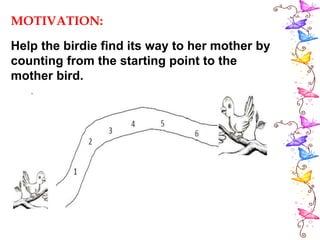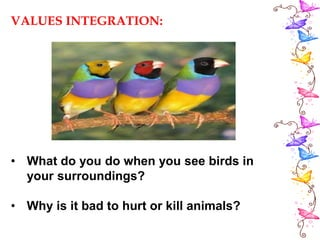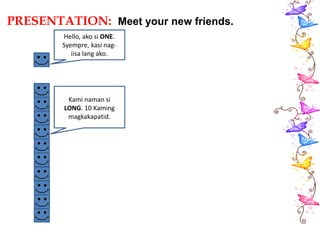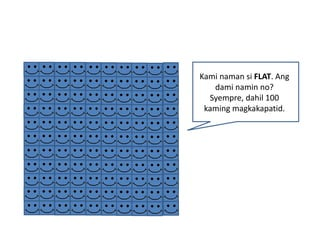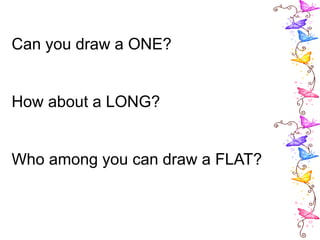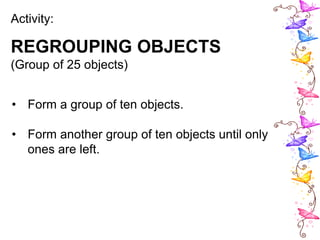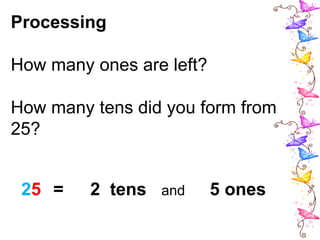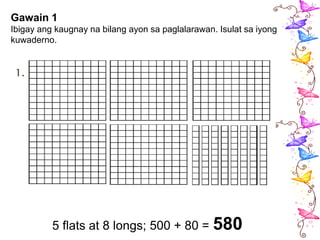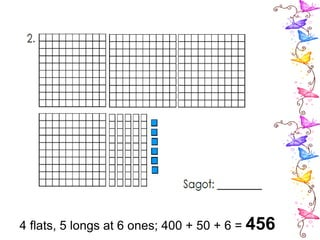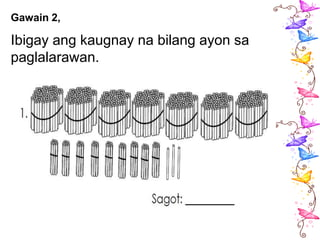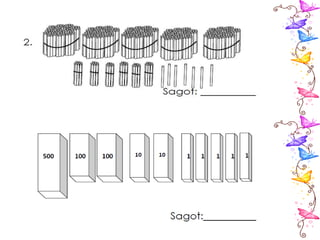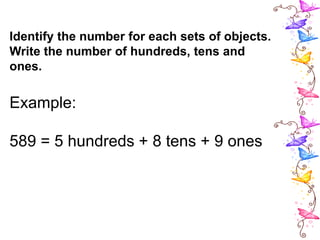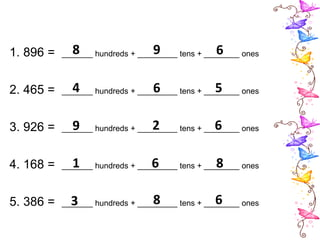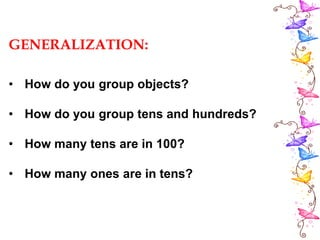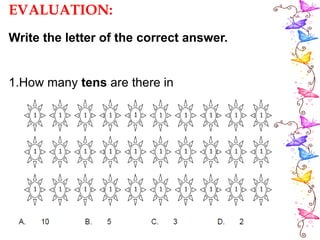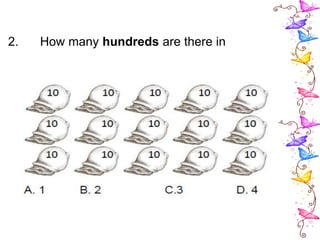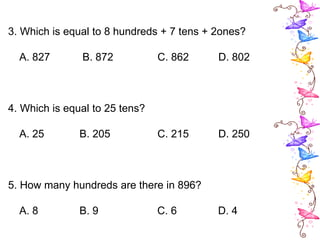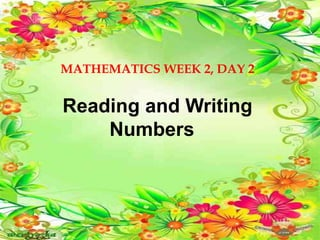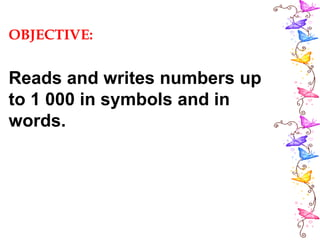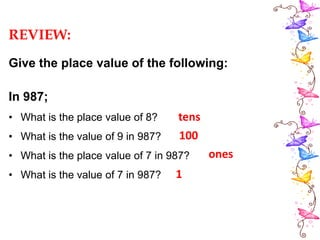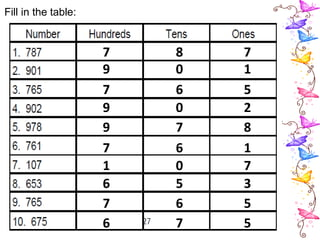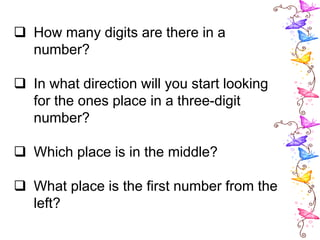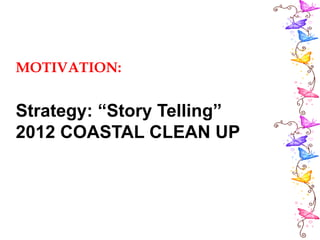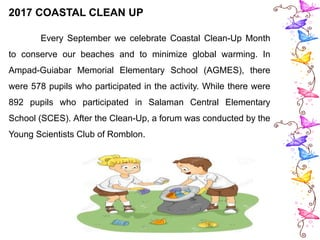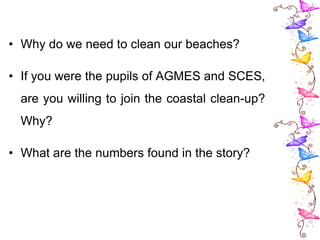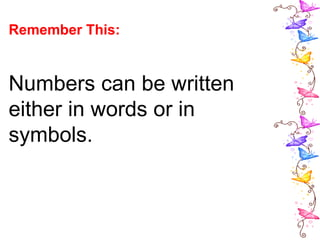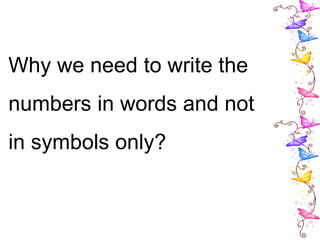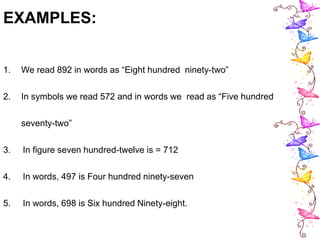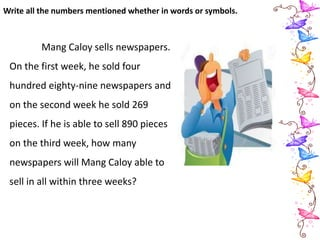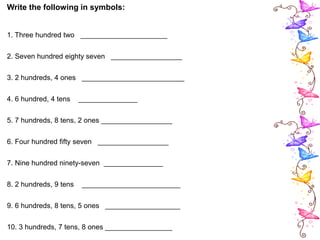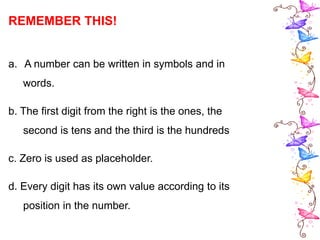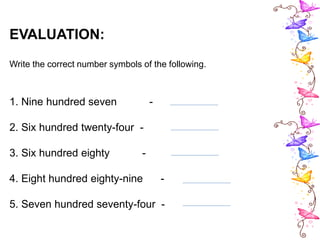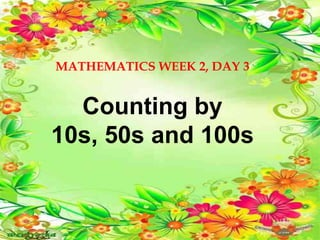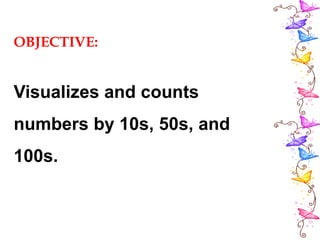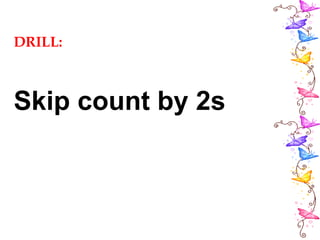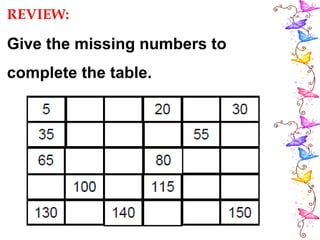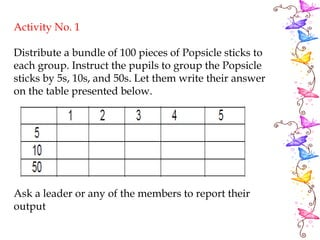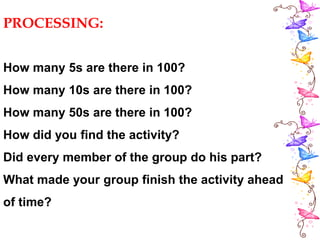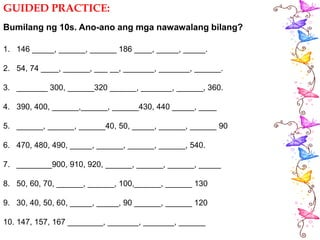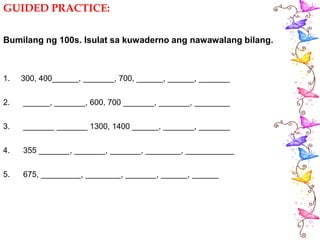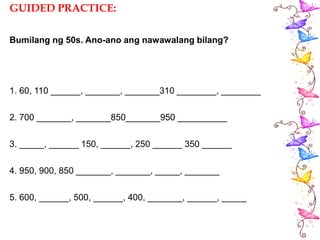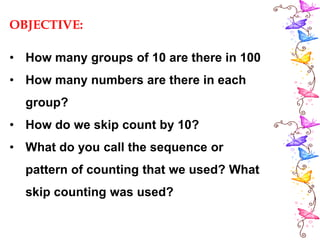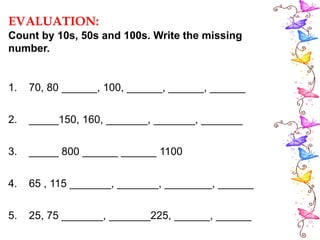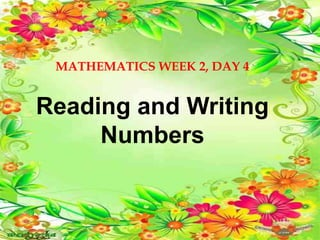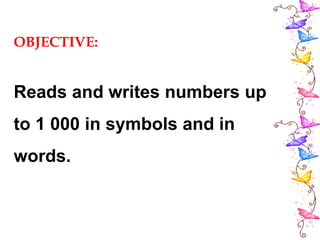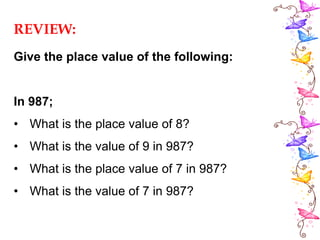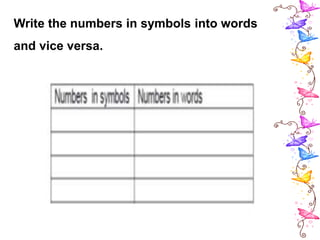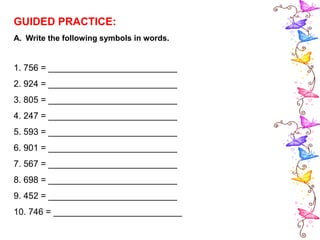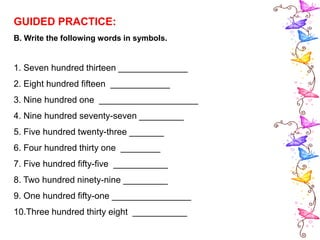1 von 61
Anzeige

1. MATHEMATICS WEEK 2, DAY 1 Counting Ones, Tens and Hundreds
2. OBJECTIVE: Groups objects in ones, tens, and hundreds.
3. DRILL: Mentally add the following: 1. 100 + 100 = 2. 10 + 10 = 3. 100 + 20 = 4. 200+80 = 5. 500+100 = 6. 800 + 10 = 7. 200 + 100 = 8. 900 + 2 = 9. 600 + 40 = 10. 700 + 12 = 200 20 120 280 600 810 300 902 640 712
4. PRE-ASSESSMENT: Give the number for each set of objects. = 543 = 192
5. = 902 = 857 = 586
6. MOTIVATION: Help the birdie find its way to her mother by counting from the starting point to the mother bird.
7. VALUES INTEGRATION: • What do you do when you see birds in your surroundings? • Why is it bad to hurt or kill animals?
8. PRESENTATION: Meet your new friends. Hello, ako si ONE. Syempre, kasi nag- iisa lang ako. Kami naman si LONG. 10 Kaming magkakapatid.
9. Kami naman si FLAT. Ang dami namin no? Syempre, dahil 100 kaming magkakapatid.
10. Can you draw a ONE? How about a LONG? Who among you can draw a FLAT?
11. Activity: REGROUPING OBJECTS (Group of 25 objects) • Form a group of ten objects. • Form another group of ten objects until only ones are left.
12. Processing How many ones are left? How many tens did you form from 25? 25 = 2 tens and 5 ones
13. Gawain 1 Ibigay ang kaugnay na bilang ayon sa paglalarawan. Isulat sa iyong kuwaderno. 5 flats at 8 longs; 500 + 80 = 580
14. 4 flats, 5 longs at 6 ones; 400 + 50 + 6 = 456
15. Gawain 2, Ibigay ang kaugnay na bilang ayon sa paglalarawan.
16. Identify the number for each sets of objects. Write the number of hundreds, tens and ones. Example: 589 = 5 hundreds + 8 tens + 9 ones
17. 1. 896 = _______ hundreds + _________ tens + ________ ones 2. 465 = _______ hundreds + _________ tens + ________ ones 3. 926 = _______ hundreds + _________ tens + ________ ones 4. 168 = _______ hundreds + _________ tens + ________ ones 5. 386 = _______ hundreds + _________ tens + ________ ones 8 9 6 4 6 5 9 2 6 1 6 8 3 8 6
18. GENERALIZATION: • How do you group objects? • How do you group tens and hundreds? • How many tens are in 100? • How many ones are in tens?
19. EVALUATION: Write the letter of the correct answer. 1.How many tens are there in
20. 2. How many hundreds are there in
21. 3. Which is equal to 8 hundreds + 7 tens + 2ones? A. 827 B. 872 C. 862 D. 802 4. Which is equal to 25 tens? A. 25 B. 205 C. 215 D. 250 5. How many hundreds are there in 896? A. 8 B. 9 C. 6 D. 4
22. MATHEMATICS WEEK 2, DAY 2 Reading and Writing Numbers
23. OBJECTIVE: Reads and writes numbers up to 1 000 in symbols and in words.
24. REVIEW: Give the place value of the following: In 987; • What is the place value of 8? • What is the value of 9 in 987? • What is the place value of 7 in 987? • What is the value of 7 in 987? tens 100 ones 1
25. Fill in the table: 7 8 7 9 0 1 7 6 5 9 0 2 9 7 8 7 6 1 1 0 7 6 5 3 7 6 5 6 7 5
26.  How many digits are there in a number?  In what direction will you start looking for the ones place in a three-digit number?  Which place is in the middle?  What place is the first number from the left?
27. MOTIVATION: Strategy: “Story Telling” 2012 COASTAL CLEAN UP
28. 2017 COASTAL CLEAN UP Every September we celebrate Coastal Clean-Up Month to conserve our beaches and to minimize global warming. In Ampad-Guiabar Memorial Elementary School (AGMES), there were 578 pupils who participated in the activity. While there were 892 pupils who participated in Salaman Central Elementary School (SCES). After the Clean-Up, a forum was conducted by the Young Scientists Club of Romblon.
29. • Why do we need to clean our beaches? • If you were the pupils of AGMES and SCES, are you willing to join the coastal clean-up? Why? • What are the numbers found in the story?
30. Remember This: Numbers can be written either in words or in symbols.
31. Why we need to write the numbers in words and not in symbols only?
32. EXAMPLES: 1. We read 892 in words as “Eight hundred ninety-two” 2. In symbols we read 572 and in words we read as “Five hundred seventy-two” 3. In figure seven hundred-twelve is = 712 4. In words, 497 is Four hundred ninety-seven 5. In words, 698 is Six hundred Ninety-eight.
33. Write all the numbers mentioned whether in words or symbols. Mang Caloy sells newspapers. On the first week, he sold four hundred eighty-nine newspapers and on the second week he sold 269 pieces. If he is able to sell 890 pieces on the third week, how many newspapers will Mang Caloy able to sell in all within three weeks?
34. Write the following in symbols: 1. Three hundred two ______________________ 2. Seven hundred eighty seven __________________ 3. 2 hundreds, 4 ones __________________________ 4. 6 hundred, 4 tens _______________ 5. 7 hundreds, 8 tens, 2 ones __________________ 6. Four hundred fifty seven __________________ 7. Nine hundred ninety-seven _______________ 8. 2 hundreds, 9 tens _________________________ 9. 6 hundreds, 8 tens, 5 ones ___________________ 10. 3 hundreds, 7 tens, 8 ones _________________
35. REMEMBER THIS! a. A number can be written in symbols and in words. b. The first digit from the right is the ones, the second is tens and the third is the hundreds c. Zero is used as placeholder. d. Every digit has its own value according to its position in the number.
36. EVALUATION: Write the correct number symbols of the following. 1. Nine hundred seven - 2. Six hundred twenty-four - 3. Six hundred eighty - 4. Eight hundred eighty-nine - 5. Seven hundred seventy-four -
37. MATHEMATICS WEEK 2, DAY 3 Counting by 10s, 50s and 100s
38. OBJECTIVE: Visualizes and counts numbers by 10s, 50s, and 100s.
39. DRILL: Skip count by 2s
40. REVIEW: Give the missing numbers to complete the table.
41. Activity No. 1 Distribute a bundle of 100 pieces of Popsicle sticks to each group. Instruct the pupils to group the Popsicle sticks by 5s, 10s, and 50s. Let them write their answer on the table presented below. Ask a leader or any of the members to report their output
42. PROCESSING: How many 5s are there in 100? How many 10s are there in 100? How many 50s are there in 100? How did you find the activity? Did every member of the group do his part? What made your group finish the activity ahead of time?
43. GUIDED PRACTICE: Bumilang ng 10s. Ano-ano ang mga nawawalang bilang? 1. 146 _____, ______, ______ 186 ____, _____, _____. 2. 54, 74 ____, ______, ___ __, _______, _______, ______. 3. _______ 300, ______320 ______, _______, ______, 360. 4. 390, 400, ______,______, ______430, 440 _____, ____ 5. ______, ______, ______40, 50, _____, ______, ______ 90 6. 470, 480, 490, _____, ______, ______, ______, 540. 7. ________900, 910, 920, ______, ______, ______, _____ 8. 50, 60, 70, ______, ______, 100,______, ______ 130 9. 30, 40, 50, 60, _____, _____, 90 ______, ______ 120 10. 147, 157, 167 ________, _______, _______, ______
44. GUIDED PRACTICE: Bumilang ng 100s. Isulat sa kuwaderno ang nawawalang bilang. 1. 300, 400______, _______, 700, ______, ______, _______ 2. ______, _______, 600, 700 _______, _______, ________ 3. _______ _______ 1300, 1400 ______, _______, _______ 4. 355 _______, _______, _______, ________, ___________ 5. 675, _________, ________, _______, ______, ______
45. GUIDED PRACTICE: Bumilang ng 50s. Ano-ano ang nawawalang bilang? 1. 60, 110 ______, _______, _______310 ________, ________ 2. 700 _______, _______850_______950 __________ 3. _____, ______ 150, ______, 250 ______ 350 ______ 4. 950, 900, 850 _______, _______, _____, _______ 5. 600, ______, 500, ______, 400, _______, ______, _____
46. OBJECTIVE: • How many groups of 10 are there in 100 • How many numbers are there in each group? • How do we skip count by 10? • What do you call the sequence or pattern of counting that we used? What skip counting was used?
47. EVALUATION: Count by 10s, 50s and 100s. Write the missing number. 1. 70, 80 ______, 100, ______, ______, ______ 2. _____150, 160, _______, _______, _______ 3. _____ 800 ______ ______ 1100 4. 65 , 115 _______, _______, ________, ______ 5. 25, 75 _______, _______225, ______, ______
48. MATHEMATICS WEEK 2, DAY 4 Reading and Writing Numbers
49. OBJECTIVE: Reads and writes numbers up to 1 000 in symbols and in words.
50. REVIEW: Give the place value of the following: In 987; • What is the place value of 8? • What is the value of 9 in 987? • What is the place value of 7 in 987? • What is the value of 7 in 987?
51. 2017 COASTAL CLEAN UP Every September we celebrate Coastal Clean-Up Month to conserve our beaches and to minimize global warming. In Ampad-Guiabar Memorial Elementary School (AGMES), there were 578 pupils who participated in the activity. While there were 892 pupils who participated in Salaman Central Elementary School (SCES). After the Clean-Up, a forum was conducted by the Young Scientists Club of Romblon.
52. • Why do we need to clean our beaches? • If you were the pupils of AGMES and SCES, are you willing to join the coastal clean-up? Why? • What are the numbers found in the story?
53. Write the numbers in symbols into words and vice versa.
54. GUIDED PRACTICE: A. Write the following symbols in words. 1. 756 = __________________________ 2. 924 = __________________________ 3. 805 = __________________________ 4. 247 = __________________________ 5. 593 = __________________________ 6. 901 = __________________________ 7. 567 = __________________________ 8. 698 = __________________________ 9. 452 = __________________________ 10. 746 = __________________________
55. GUIDED PRACTICE: B. Write the following words in symbols. 1. Seven hundred thirteen ______________ 2. Eight hundred fifteen ____________ 3. Nine hundred one ____________________ 4. Nine hundred seventy-seven _________ 5. Five hundred twenty-three _______ 6. Four hundred thirty one ________ 7. Five hundred fifty-five ___________ 8. Two hundred ninety-nine _________ 9. One hundred fifty-one ________________ 10.Three hundred thirty eight ___________
56. REMEMBER THIS! a. A number can be written in symbols and in words. b. The first digit from the right is the ones, the second is tens and the third is the hundreds c. Zero is used as placeholder. d. Every digit has its own value according to its position in the number.
Anzeige